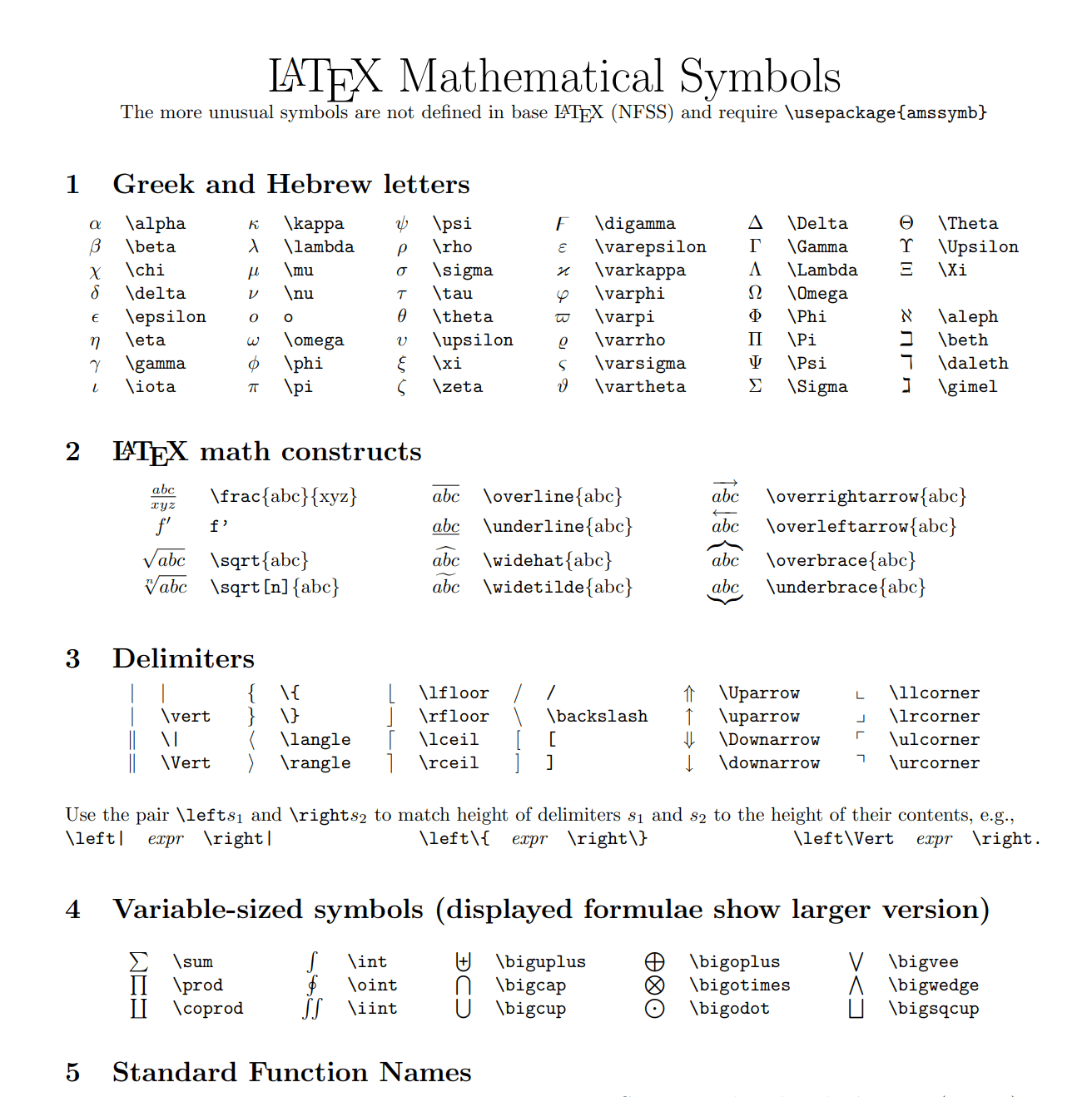# 使用Overleaf在毕业论文中插入算法伪代码，高效美观

### 【前言】去年的这个时候，一边准备考研复试，一边撰写本科毕设论文，读了很多论文，惊叹于其美观的伪代码算法，所以在之前的教程中教大家使用Aurora在Word中插入伪代码，具体可以看使用Aurora在Word中插入算法伪代码教程！！！亲测有效！！！写论文必备https://blog.csdn.net/jucksu/article/details/116307244效果如图所示（附图是本科毕设当中的K-Means聚类算法伪代码），不算很差但不是很美观，包括一些下标，公式，语法，编辑器反应慢，编程体验差，相关参考资料少等方面的缺陷。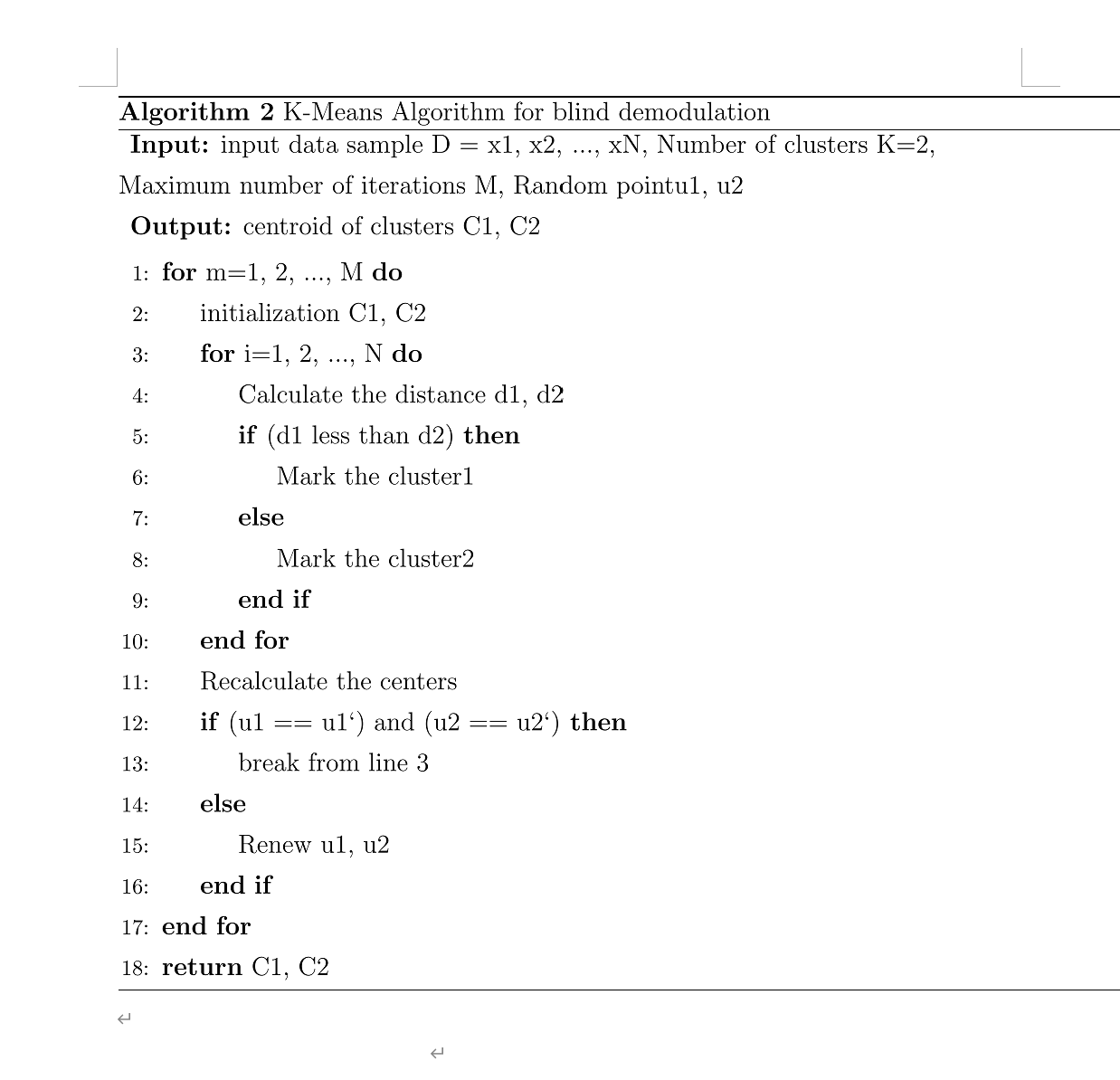## 1. 打开Overleaf网站，需要注册一个账号，一般国内的邮箱是注册不了的，可以使用ORCID邮箱注册，具体方法就不细讲了，到时候登录也是使用ORCID邮箱。### 注册完成后，使用ORCID邮箱登录（切记！！）如图：## 2. 登录后，在overleaf左侧导航栏创建新项目，选择空白项目创建即可，如图示：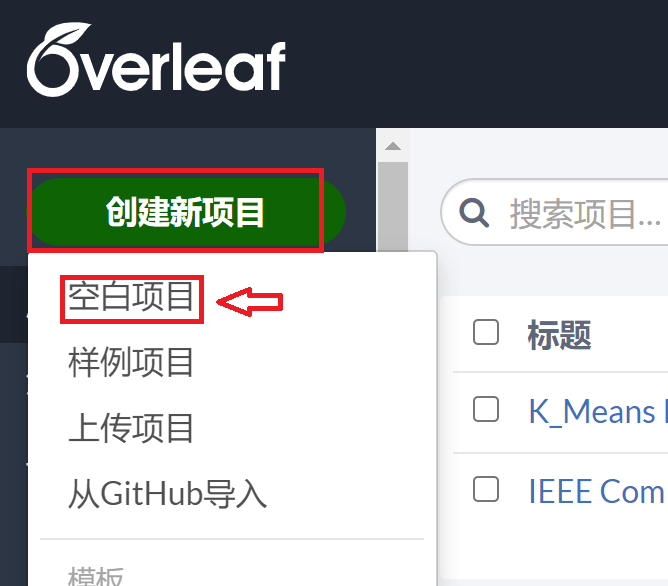## 3. 选择菜单，设置下的编译器中选择XeLaTeX，如图所示：## 4. 然后在左侧的编程区域复制以下代码：

%在菜单中，编译器选择XeLaTex

\documentclass[11pt]{ctexart}
\usepackage[top=2cm, bottom=2cm, left=2.5cm, right=2.5cm]{geometry} %定义页边距
\usepackage{algorithm}
\usepackage{algorithmicx}
\usepackage{algpseudocode}
\usepackage{amsmath} %数学公式
\usepackage[UTF8]{ctex} %输出中文
\floatname{algorithm}{Algorithm} %算法
\renewcommand{\algorithmicrequire}{\textbf{Input:}} %输入
\renewcommand{\algorithmicensure}{\textbf{Output:}} %输出

\begin{document}
\renewcommand{\thealgorithm}{2} %这里用来定义算法1，算法2等
\begin{algorithm}
\caption{K-Means聚类盲均衡算法} %标题
\begin{algorithmic} %每行显示行号，1表示每1行进行显示
\Require 输入样本集$D$ = \{$x_1,x_2,...,x_N$\},分簇数$K=2$,最大迭代次数为$M$,从分簇样本中随机选取两点\{$u_1$,$u_2$\}作为初始质心
\Ensure 样本分簇质心\{$C_1$,$C_2$\}
\For{$m = 1 \to M$} //$m$表示迭代次数
\State $C_1 \Leftarrow \emptyset, C_2 \Leftarrow \emptyset$ //初始化各簇
\For{$i = 1,2,...,N$}     //$i$表示样本集编号
\State $d_{i1} \Leftarrow {\Vert x_i-u_1 \Vert}^2$, $d_{i2} \Leftarrow {\Vert x_i-u_2 \Vert}^2$ //计算$x_i$到两质心的欧式距离
\If {$d_{i1} \leq d_{i2}$}
\State $C_1 \Leftarrow C_1 \cup \{x_i\}$ //将$x_i$划分到相应的簇
\Else
\State $C_2 \Leftarrow C_2 \cup \{x_i\}$ %有时候需要用\来转译
\EndIf
\EndFor
\State $\tilde{u_1} \Leftarrow \frac{1}{\vert C_1 \vert}\sum_{x \in C_1} x$, $\tilde{u_2} \Leftarrow \frac{1}{\vert C_2 \vert}\sum_{x \in C_2} x$ //重新计算各簇质心
\If{$(\tilde{u_1} == u_1) && (\tilde{u_2} == u_2$} //各簇质心未改变，跳出循环
\State \textbf{break} from line 3 %\textbf为加粗
\Else
\State $u_1 \Leftarrow \tilde{u_1}, u_2 \Leftarrow \tilde{u_2}$ //更新各簇质心
\EndIf
\EndFor
\State \Return $C_1, C_2$ //输出结果
\end{algorithmic}
\end{algorithm}
\end{document}

### 点击右侧的编译，如图所示：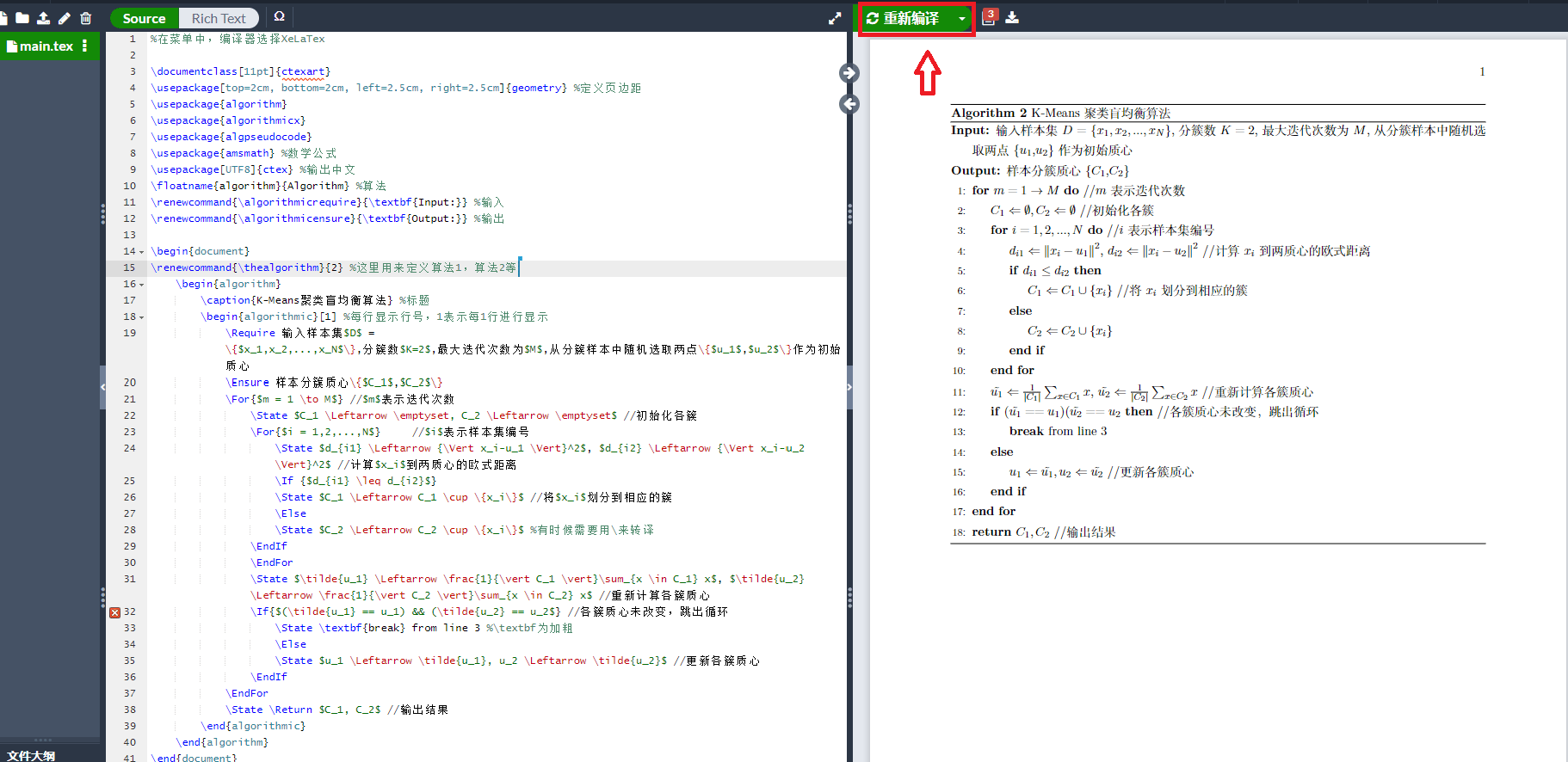### 最终的效果图如图：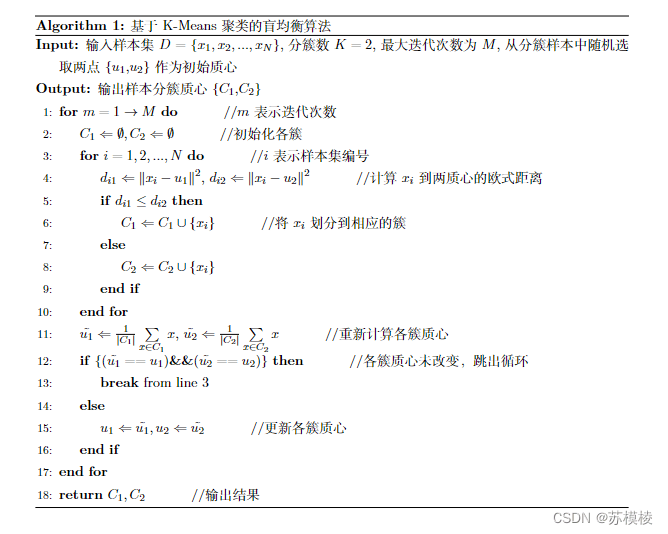## 5. 这里给大家安利一个写LaTex数学公式大全，LATEX Mathematical Symbols，非常全面，PDF可以下载自己保存，然后通过查表来进行LaTex编程，如图所示：